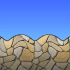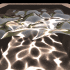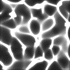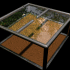## Archive for the 'waves' Category

02
Nov
07

### Rayleigh Surface WavesRayleigh Surface Waves are associated with ocean waves and earthquakes.

Here is some Mathematica code:

```(* runtime: 0.2 second *) omega = 2 Pi; k = 7; nu = 0.33; n = 0.175; xi = Sqrt[(0.5 - nu)/(1 - nu)]; q = k Sqrt[1 - n^2 xi^2]; s = k Sqrt[1 - n^2]; Do[grid = Table[{x + k (Exp[q y] - 2 q s Exp[s y]/(s^2 + k^2)) Sin[omega t - k x], y + q (Exp[q y] - 2 k^2 Exp[s y]/(s^2 + k^2)) Cos[omega t - k x]}, {x, 0, 2, 0.1}, {y, -1, 0, 0.1}]; Show[Graphics[{Map[Line, grid], Map[Line, Transpose[grid]]}, AspectRatio -> Automatic, PlotRange -> {{-0.1, 2.1}, {-1.1, 0.25}}]], {t, 0, 1, 0.05}];```

05
May
07

### 2D Wave-Particle Simulations2D Wave-Particle Simulations (Mathematica 4.2, 1/15/05; C++ version: 5/16/07) The left animation shows the quantum mechanical probabilty distribution of an electron as it passes through two narrow slits. The electron itself is much smaller than its probability distribution cloud, which is dispersed over a large area, creating an interference pattern. As soon as the actual location of the electron itself is detected, then its probability distribution cloud will become small again. The color represents the complex phase. The following Mathematica code uses the Crank-Nicolson method with time-splitting to solve the Schrödinger wave equation:
```(* runtime: 10 seconds, V is the potential energy *) << LinearAlgebra`Tridiagonal`; n = 55; dx = 1.0/(n - 1); hbar = m = 1; dt = m dx^2/hbar; a = I hbar/dt; b = hbar^2/(4 m dx^2); blist = Table[b, {n - 1}]; psi = Table[Module[{p = {x - 0.25, y - 0.5}}, Exp[I {100, 0}.p - p.p/(2 0.14^2)]], {y, 0, 1, dx}, {x, 0, 1, dx}]; V = Table[If[0.48 < x < 0.52 && ! (0.4 < y < 0.475 || 0.525 < y < 0.6), 10000, 0], {y, 0, 1, dx}, {x, 0, 1, dx}]; Do[psi = Table[TridiagonalSolve[blist, a - 2b - V[[All, j]]/2, blist, (a + 2b + V[[All, j]]/2)psi[[All, j]] - b Table[psi[[i, Min[n, j + 1]]] + psi[[i, Max[1, j - 1]]], {i, 1, n}]], {j, 1, n}]; psi = Table[TridiagonalSolve[blist, a - 2b - V[[i,All]]/2, blist, (a + 2b + V[[i, All]]/2)psi[[All, i]] - b Table[psi[[j, Min[n, i + 1]]] + psi[[j, Max[1, i - 1]]], {j, 1, n}]], {i, 1, n}]; Show[Graphics[RasterArray[Map[(x = Min[Abs[#]^2, 1];Hue[Arg[#]/(2Pi), Min[1, 2(1 - x)], Min[1, 2x]]) &, psi + V, {2}]], AspectRatio -> 1]], {40}];```In case you’re wondering how TridiagonalSolve works:
`TridiagonalSolve[a_, b_, c_, r_] := Module[{n = Length[r], p, q}, p = q =Table[0, {n}]; {p[], q[]} = {c[], r[]}/b[]; Do[{p[[i]], q[[i]]} = {If[i < n, c[[i]], 0], r[[i]] - a[[i - 1]]q[[i - 1]]}/(b[[i]] - a[[i - 1]]p[[i - 1]]), {i, 2, n}]; Do[q[[i]] -= p[[i]]q[[i + 1]], {i, n - 1, 1, -1}]; q];`

22
Apr
06

### Water CausticsThis water-like surface was generated in Mathematica using frequency filtered random noise, and then it was raytraced in POV-Ray and water caustics were added using Henrik Jensen’sphoton mapping technique. The smooth_triangle command was used for phong normal interpolation of the water’s surface.

Link: Water Caustics Generator – C++ program by Kjell Andersson, uses Heron’s Formula to calculate the caustics

29
Jul
05

### Water Ripple SimulationHere is a simple water ripple simulation showing single slit wave diffraction. The following Mathematica code solves the wave equation with damping using the finite difference method. You can read more about this algorithm on Hugo Elias’ website. (Note: technically this simulation should use Neumann boundary conditions but I decided it was simplier to demonstrate using Dirichlet boundary conditions).

`(* runtime: 18 seconds, c is the wave speed and b is a damping factor *) n = 64; c = 1; b = 5; dx = 1.0/(n - 1); Courant = Sqrt[2.0]/2;dt = Courant dx/c; z1 = z2 = Table[0.0, {n}, {n}]; Do[{z1, z2} = {z2, z1}; z1[[n/2, n/4]] = Sin[16Pi t]; Do[If[0.45 < i/n < 0.55 || ! (0.48 < j/n < 0.52), z2[[i, j]] = (2(1 - 2Courant^2)z1[[i, j]] + Courant^2(z1[[i - 1, j]] + z1[[i + 1, j]] + z1[[i, j - 1]] + z1[[i, j + 1]]))/(1 + b dt) - z2[[i, j]]], {i, 2, n - 1}, {j, 2, n - 1}]; ListPlot3D[z1, Mesh -> False, PlotRange -> {-1, 1}], {t, 0, 1, dt}];`

24
Aug
04

### Wave InterferenceThis interference pattern is the superposition of two wave sources, such as light passing through two narrow slits.
```(* runtime: 9 seconds *) Do[DensityPlot[Sin[2Pi(Sqrt[x^2 + (y - 3)^2] + t)] + Sin[2Pi(Sqrt[x^2 + (y + 3)^2] + t)], {x, 0, 16}, {y, -8, 8}, PlotPoints -> 275, Mesh -> False, Frame -> False], {t, 0, 1, 0.1}];```

## Welcome !

You will find here some of my favorite hobbies and interests, especially science and art.

I hope you enjoy it!

Subscribe to theRSS feed to stay informed when I publish something new here.

I would love to hear from you! Please feel free to send me an email : bugman123-at-gmail-dot-com

## TopicsBerna Blalack on Magnetic Pendulum Strange…Daan on Magnetic Pendulum Strange…Sebastian Schepis on Diffusion Limited Aggregation…mohammad_andito on CFM56-5 Turbofan Jet Engi…SasQ on Magnetic Field of a Solen… OUPblog » Blog… on Diamond Light Dispersion Complex Roots on Polynomial Roots Joukowski airfoils |… on Joukowski AirfoilKarim Alame on Flapping WingREJISH J on Joukowski Airfoil SOLINOID | Materials… on Magnetic Field of a Solen…Emanuele on 4D “Squarry” Julia…Emanuele on Hydrogen Electron Orbital Prob…Tim on Mandelbrot Set Pickover S…khankasi1 on 4D Quaternion Mandelbrot …